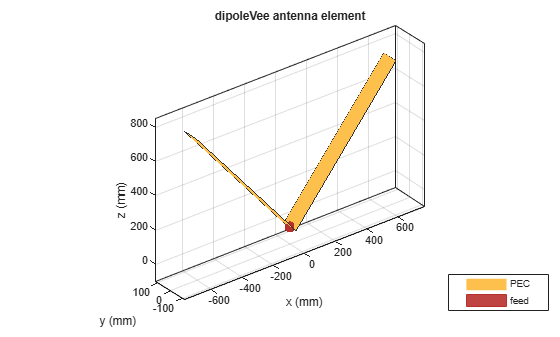# dipoleVee

Create V-dipole antenna

## Description

The `dipoleVee` object is a planar V-dipole antenna in the xy- plane.

The width of the dipole is related to the circular cross-section by the equation

`$w=2d=4r$`

, where:

• d is the diameter of equivalent cylindrical pole

• r is the radius of equivalent cylindrical pole

For a given cylinder radius, use the `cylinder2strip` utility function to calculate the equivalent width. The V-dipole antenna is bent around the feed point. The default V-dipole is center-fed and is in the xy- plane. The feed point of the V-dipole antenna coincides with the origin.## Creation

### Syntax

``dv = dipoleVee``
``dv = dipoleVee(Name,Value)``

### Description

``` `dv = dipoleVee` creates a half-wavelength V-dipole antenna.```

example

``` `dv = dipoleVee(Name,Value)` creates a half-wavelength V-dipole antenna, with additional properties specified by one or more name-value pair arguments. `Name` is the property name and `Value` is the corresponding value. You can specify several name-value pair arguments in any order as `Name1`, `Value1`, `...`, `NameN`, `ValueN`. Properties not specified retain their default values.```

## Properties

expand all

Length of two arms, specified as a two-element vector in meters. By default, the arm lengths are chosen for an operating frequency of 75 MHz.

Example: `'ArmLength',[1,3]`

Data Types: `double`

V-dipole arm width, specified as a scalar in meters.

Note

Dipole width should be less than `Total Arm Length`/5 and greater than `Total Arm Length`/1001. 

Example: `'Width',0.05`

Data Types: `double`

Angle made by two arms about xy- plane, specified as a two-element vector in degrees.

Example: `'ArmElevation',[55 35]`

Data Types: `double`

Type of the metal used as a conductor, specified as a metal material object. You can choose any metal from the `MetalCatalog` or specify a metal of your choice. For more information, see `metal`. For more information on metal conductor meshing, see Meshing.

Example: ```m = metal('Copper'); 'Conductor',m```

Example: ```m = metal('Copper'); ant.Conductor = m```

Lumped elements added to the antenna feed, specified as a lumped element object. For more information, see `lumpedElement`.

Example: `'Load',lumpedelement`. `lumpedelement` is the object for the load created using `lumpedElement`.

Example: ```dv.Load = lumpedElement('Impedance',75)```

Tilt angle of the antenna in degrees, specified as a scalar or vector. For more information, see Rotate Antennas and Arrays.

Example: `90`

Example: `Tilt=[90 90]`,`TiltAxis=[0 1 0;0 1 1]` tilts the antenna at 90 degrees about the two axes defined by the vectors.

Data Types: `double`

Tilt axis of the antenna, specified as one of these values:

• Three-element vector of Cartesian coordinates in meters. In this case, each coordinate in the vector starts at the origin and lies along the specified points on the x-, y-, and z-axes.

• Two points in space, specified as a 2-by-3 matrix corresponding to two three-element vectors of Cartesian coordinates. In this case, the antenna rotates around the line joining the two points.

• `"x"`, `"y"`, or `"z"` to describe a rotation about the x-, y-, or z-axis, respectively.

Example: `[0 1 0]`

Example: `[0 0 0;0 1 0]`

Example: `"Z"`

Data Types: `double` | `string`

## Object Functions

 `show` Display antenna, array structures, shapes, or platform `info` Display information about antenna or array `axialRatio` Axial ratio of antenna `beamwidth` Beamwidth of antenna `charge` Charge distribution on antenna or array surface `current` Current distribution on antenna or array surface `design` Design prototype antenna or arrays for resonance around specified frequency or create AI-based antenna from antenna catalog objects `efficiency` Radiation efficiency of antenna `EHfields` Electric and magnetic fields of antennas or embedded electric and magnetic fields of antenna element in arrays `impedance` Input impedance of antenna or scan impedance of array `mesh` Mesh properties of metal, dielectric antenna, or array structure `meshconfig` Change meshing mode of antenna, array, custom antenna, custom array, or custom geometry `optimize` Optimize antenna or array using SADEA optimizer `pattern` Plot radiation pattern and phase of antenna or array or embedded pattern of antenna element in array `patternAzimuth` Azimuth plane radiation pattern of antenna or array `patternElevation` Elevation plane radiation pattern of antenna or array `rcs` Calculate and plot radar cross section (RCS) of platform, antenna, or array `returnLoss` Return loss of antenna or scan return loss of array `sparameters` Calculate S-parameters for antennas and antenna arrays `vswr` Voltage standing wave ratio (VSWR) of antenna or array element

## Examples

collapse all

Create and view a center-fed V-dipole that has 50 degree arm angles.

`dv = dipoleVee(ArmElevation=[50 50])`
```dv = dipoleVee with properties: ArmLength: [1 1] ArmElevation: [50 50] Width: 0.1000 Conductor: [1x1 metal] Tilt: 0 TiltAxis: [1 0 0] Load: [1x1 lumpedElement] ```
`show(dv)`Calculate the impedance of a V-dipole antenna over the frequency range of 50 MHz - 100 MHz.

```dv = dipoleVee('ArmElevation',[50 50]); impedance(dv,linspace(50e6,100e6,51))```Balanis, C.A. Antenna Theory: Analysis and Design. 3rd Ed. New York: Wiley, 2005.

 Volakis, John. Antenna Engineering Handbook. 4th Ed. New York: McGraw-Hill, 2007.